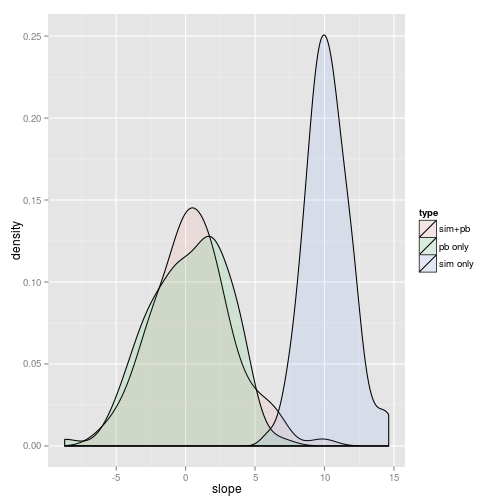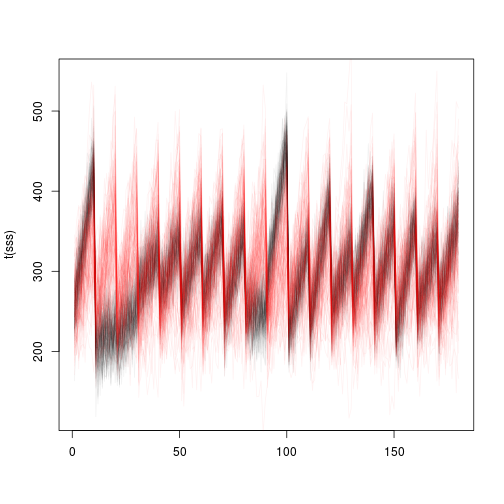# Prediction and simulation in lme4

## Fixed effects

### Predicted value

Pretty easy: use model.matrix() to compute new $$X$$, compute $$X \beta$$. Add offset if necessary. Make sure to handle na.action() appropriately.

### Variance due to uncertainty in fixed effect parameters

Also fairly easy: if $$V$$ is the variance-covariance matrix of $$\beta$$ (as in vcov(.)), then $$X V X^T$$ gives us the variance-covariance matrix of the predictions; extract the diagonal to get the variances only.

### Marginalizing

For lsmeans-like capability, we need to be able to specify how we are marginalizing. This can be done by resetting contrasts, but it might also be OK to do it by averaging predictions. There are two ways to marginalize – unweighted or population-weighted. Since these are linear combinations we should be able to propagate the variance due to uncertainty in fixed effects …

## Random effects

Things get a little bit more complicated here. In principle we can produce four different kinds of predictions: (1) conditional on $$b$$; (2) population-level (force $$b=0$$); (3) marginal across the distribution (unweighted); (4) marginal across the observed distribution of the data (weighted).

If we want to simulate,

## Conditional distribution

If we want to get prediction rather than confidence intervals, we also need to add randomness due to the conditional distribution of the responses. In the LMM case this is just adding a $$\text{Normal}(0,\hat \sigma^2)$$ deviate where $$\hat \sigma^2$$ is the residual variance. In the GLMM case we have to draw from the appropriate conditional distribution. In principle we ought to be able to extract information from the family object to do this – then we could simulate for any user-specified family rather than having to hard-code – but in practice it doesn't quite work. Maybe we can figure something out.

## Packages that do stuff like this

effects, contrasts (car?), lsmeansrockchalk ?

library(lme4)

## Loading required package: lattice

fm1 <- lmer(Reaction ~ Days + (Days | Subject), sleepstudy)
fm0 <- update(fm1, . ~ . - Days)


## Simulation examples

These examples use capabilities in the development/predsim branches.

S1 <- arm::sim(fm1)  ## 100 sims
S0 <- arm::sim(fm0)  ## 100 sims
fvec1 <- fvec2 <- numeric(100)
for (i in 1:100) {
ss1 <- simulate(fm0, newparams = list(beta = S0@fixef[i]))[]
ss2 <- simulate(fm0)[]
fvec1[i] <- getME(refit(fm1, ss1), "beta")
fvec2[i] <- getME(refit(fm1, ss2), "beta")
}

plot(density(fvec1))
lines(density(fvec2))fdat <- rbind(data.frame(slope = fvec1, type = "sim+pb"), data.frame(slope = fvec2,
type = "pb only"), data.frame(slope = S1@fixef[, 2], type = "sim only"))

library(ggplot2)
ggplot(fdat, aes(x = slope, fill = type)) + geom_density(alpha = 0.1)sss <- do.call(rbind, simulate(fm1, use.u = TRUE, nsim = 100))
matplot(t(sss), type = "l", lty = 1, col = adjustcolor("black", 0.05))
sss2 <- do.call(rbind, simulate(fm1, use.u = FALSE, nsim = 100))
matlines(t(sss2), type = "l", lty = 1, col = adjustcolor("red", 0.05))In general want to consider

* what we can do with sim(), simulate(), refit()
* various sampling approximations
* can we sample from a density function approximated by the likelihood profile?
* importance sampling based on arm::sim()?  (Does arm::sim() do resampling of theta yet?)
* value of having newparms, newdata in both predict and simulate